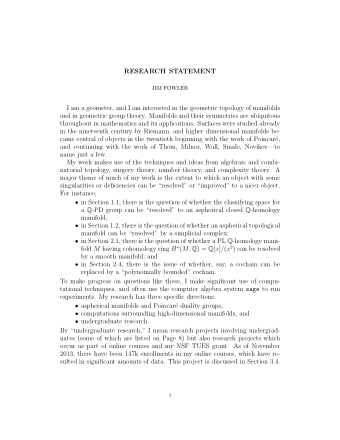# Research Statement

I am a geometer, and I am interested in the geometric topology of manifolds and in geometric group theory. Manifolds and their symmetries are ubiquitous throughout in mathematics and its applications. Surfaces were studied already in the nineteenth century by Riemann, and higher dimensional manifolds became central of objects in the twentieth beginning with the work of Poincaré, and continuing with the work of Thom, Milnor, Wall, Smale, Novikov—to name just a few.

Broadly speaking, my work focuses on aspherical manifolds, Poincaré duality groups, and computations for high-dimensional manifolds. My work makes use of the techniques and ideas from algebraic and combinatorial topology, surgery theory, number theory, and complexity theory. Specifically, a major theme of much of my work is the extent to which a geometric object with some singularities or deficiencies can be “resolved” or “improved” to a nicer object. For instance,

• a paper of mine shows that there are aspherical topological $n$-manifolds (for $n \geq 6$) that cannot be “resolved” by a simplicial complex, i.e., they are not triangulable;
• there is a PL $\mathbb{Q}$-homology $4n$-manifold $M$ having cohomology ring $H^\star(M;\mathbb{Q}) = \mathbb{Q}[x]/(x^3)$, which I have shown cannot be improved to a smooth manifold unless $n = 2^a + 2^b$;
• my thesis addressed the question of whether the classifying space for a $\mathbb{Q}$-PD group can be “resolved” by an aspherical closed $\mathbb{Q}$-homology manifold; and finally
• I have studied the extent to which a cochain can be replaced by a polynomially-bounded cochain, culminating in my developing polynomially-bounded variant of algebraic $K$-theory (see MR2962981).

To make progress on questions like these, I make significant use of computational techniques, and often use the computer algebra system Sage to run experiments.

In addition to my geometric projects, I also work on adaptive learning. As of November 2013, there have been 147k student enrollments in my online courses, which has lead to millions of correct (and incorrect) answers being submitted. The resulting data is now being used to train a hidden Markov model that I designed. The Transforming Undergraduate Education in STEM (TUES) Type 1 award for my proposal DUE–1245433 will contribute to this project.#### Download as PDF

A longer version of my research statement is available as a PDF.